Learn data science and machine learning by building real-world projects on Jovian

## SOLAR POWER GENERATION!!!!!!!

Solar power is the conversion of sun radiation into electricity through the use of solar photovoltaic cells. This conversion takes place in the solar cell by photovoltaic effect. As said by many experts that the amount of solar energy reaching the earth is more than 10000 times the current energy consumption by man.

Also, the power created by solar is sufficient for one year for the entire planet, if we could convert the 100 percent of the solar energy into electricity in one hour.

i have learning all these innovative from #"Data Analysis with Python: Zero to Pandas" #Data Analysis with Python: Zero to Pandas" is a practical, beginner-friendly and coding-focused introduction to data analysis covering the basics of Python, Numpy, Pandas, data visualization and exploratory data analysis.

In :
``!pip install jovian opendatasets --upgrade --quiet``
In :
``dataset_url = 'https://www.kaggle.com/anikannal/solar-power-generation-data' ``
In :
``````import opendatasets as od
``````
```Please provide your Kaggle credentials to download this dataset. Learn more: http://bit.ly/kaggle-creds Your Kaggle username: akanshasaini Your Kaggle Key: ········ ```
`100%|██████████| 1.90M/1.90M [00:00<00:00, 159MB/s]`
```Downloading solar-power-generation-data.zip to ./solar-power-generation-data ```
``` ```

In :
``data_dir = './solar-power-generation-data'``
In :
``````import os
os.listdir(data_dir)
``````
Out:
``````['Plant_1_Generation_Data.csv',
'Plant_2_Weather_Sensor_Data.csv',
'Plant_1_Weather_Sensor_Data.csv',
'Plant_2_Generation_Data.csv']``````

Let us save and upload our work to Jovian before continuing.

In :
``project_name = "zerotopandas-course-project-starter-solar power generation"``
In :
``!pip install jovian --upgrade -q``
In :
``import jovian``
In :
``jovian.commit(project= "solar-power-generation")``
```[jovian] Attempting to save notebook.. [jovian] Updating notebook "akanshasaini888/solar-power-generation" on https://jovian.ml/ [jovian] Uploading notebook.. [jovian] Capturing environment.. [jovian] Committed successfully! https://jovian.ml/akanshasaini888/solar-power-generation ```
Out:
``'https://jovian.ml/akanshasaini888/solar-power-generation'``

## Data Preparation and Cleaning

In :
``````!pip install jovian --upgrade --quiet

``````
In :
``!pip install numpy seaborn pandas matplotlib``
```Requirement already satisfied: numpy in /srv/conda/envs/notebook/lib/python3.8/site-packages (1.19.1) Requirement already satisfied: seaborn in /srv/conda/envs/notebook/lib/python3.8/site-packages (0.10.1) Requirement already satisfied: pandas in /srv/conda/envs/notebook/lib/python3.8/site-packages (1.1.2) Requirement already satisfied: matplotlib in /srv/conda/envs/notebook/lib/python3.8/site-packages (3.3.0) Requirement already satisfied: scipy>=1.0.1 in /srv/conda/envs/notebook/lib/python3.8/site-packages (from seaborn) (1.5.2) Requirement already satisfied: python-dateutil>=2.7.3 in /srv/conda/envs/notebook/lib/python3.8/site-packages (from pandas) (2.8.1) Requirement already satisfied: pytz>=2017.2 in /srv/conda/envs/notebook/lib/python3.8/site-packages (from pandas) (2020.1) Requirement already satisfied: kiwisolver>=1.0.1 in /srv/conda/envs/notebook/lib/python3.8/site-packages (from matplotlib) (1.2.0) Requirement already satisfied: cycler>=0.10 in /srv/conda/envs/notebook/lib/python3.8/site-packages (from matplotlib) (0.10.0) Requirement already satisfied: pyparsing!=2.0.4,!=2.1.2,!=2.1.6,>=2.0.3 in /srv/conda/envs/notebook/lib/python3.8/site-packages (from matplotlib) (2.4.7) Requirement already satisfied: pillow>=6.2.0 in /srv/conda/envs/notebook/lib/python3.8/site-packages (from matplotlib) (7.2.0) Requirement already satisfied: six>=1.5 in /srv/conda/envs/notebook/lib/python3.8/site-packages (from python-dateutil>=2.7.3->pandas) (1.15.0) ```
In :
``````import numpy as np
import pandas as pd
import seaborn as sns
import matplotlib.pyplot as plt
import jovian
%matplotlib inline
``````
In :
``````solar_raw_df=pd.read_csv(data_dir +"/Plant_1_Generation_Data.csv")

``````
In :
``````solar_raw_df

``````
Out:
In :
``````solar_raw_df.info()

``````
```<class 'pandas.core.frame.DataFrame'> RangeIndex: 68778 entries, 0 to 68777 Data columns (total 7 columns): # Column Non-Null Count Dtype --- ------ -------------- ----- 0 DATE_TIME 68778 non-null object 1 PLANT_ID 68778 non-null int64 2 SOURCE_KEY 68778 non-null object 3 DC_POWER 68778 non-null float64 4 AC_POWER 68778 non-null float64 5 DAILY_YIELD 68778 non-null float64 6 TOTAL_YIELD 68778 non-null float64 dtypes: float64(4), int64(1), object(2) memory usage: 3.7+ MB ```
In :
``solar_raw_df.describe()``
Out:
In :
``solar_raw_df.shape``
Out:
``(68778, 7)``
In :
``````## let find the nunique values in the data frame,it ignores the nan values

solar_raw_df.nunique()
``````
Out:
``````DATE_TIME       3158
PLANT_ID           1
SOURCE_KEY        22
DC_POWER       32909
AC_POWER       32686
DAILY_YIELD    29900
TOTAL_YIELD    37267
dtype: int64``````
In :
``````## We check for Null values using isnull() function

solar_raw_df.isnull().sum()``````
Out:
``````DATE_TIME      0
PLANT_ID       0
SOURCE_KEY     0
DC_POWER       0
AC_POWER       0
DAILY_YIELD    0
TOTAL_YIELD    0
dtype: int64``````
In :
``````## this is the visual representation of above

sns.heatmap(solar_raw_df.isnull())
``````
Out:
``<AxesSubplot:>``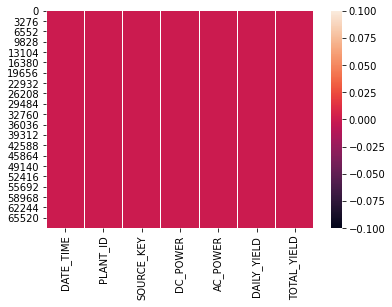In :
``nf_df=solar_raw_df.copy()``
In :
``nf_df``
Out:
In :
``````##  i have decided to drop the columns,
nf_df=solar_raw_df.copy()

nf_df.drop(['DC_POWER','AC_POWER'],axis=1,inplace=True)

nf_df
``````
Out:
In :
``import jovian``
In :
``jovian.commit()``
```[jovian] Attempting to save notebook.. [jovian] Updating notebook "akanshasaini888/solar-power-generation" on https://jovian.ml/ [jovian] Uploading notebook.. [jovian] Capturing environment.. [jovian] Committed successfully! https://jovian.ml/akanshasaini888/solar-power-generation ```
Out:
``'https://jovian.ml/akanshasaini888/solar-power-generation'``

## Exploratory Analysis and Visualization

In :
``````import seaborn as sns
import matplotlib
import matplotlib.pyplot as plt
%matplotlib inline

sns.set_style('darkgrid')
matplotlib.rcParams['font.size'] = 14
matplotlib.rcParams['figure.figsize'] = (9, 5)
matplotlib.rcParams['figure.facecolor'] = '#00000000'``````

TODO - Explore one or more columns by plotting a graph below, and add some explanation about it

In :
``sns.barplot(solar_raw_df.DC_POWER)``
Out:
``<AxesSubplot:xlabel='DC_POWER'>``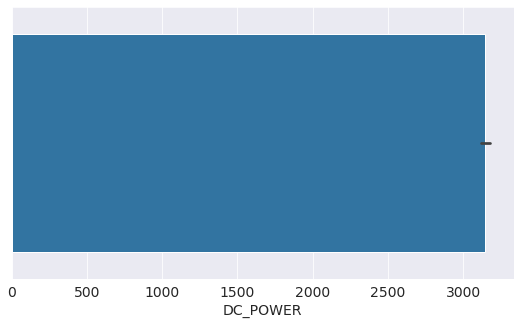In :
``nf_df['DAILY_YIELD'].value_counts()``
Out:
``````0.000000       18696
5803.000000       66
8435.000000       64
5965.000000       62
8273.000000       57
...
5525.428571        1
1596.833333        1
2879.125000        1
2126.857143        1
76.250000          1
Name: DAILY_YIELD, Length: 29900, dtype: int64``````
In :
``sns.barplot(solar_raw_df.TOTAL_YIELD)``
Out:
``<AxesSubplot:xlabel='TOTAL_YIELD'>``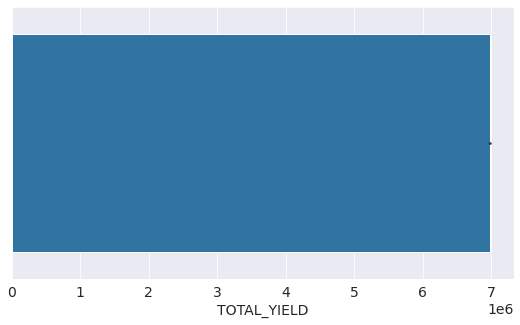In :
``sns.pairplot(nf_df);``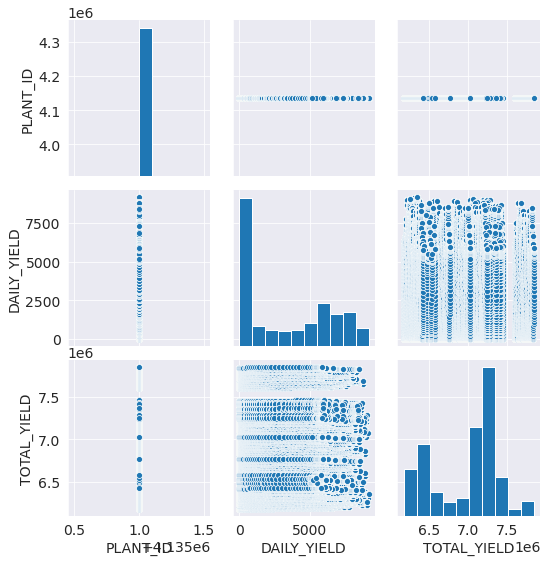In :
``````nf_df.corr()

``````
Out:
In :
``plt.hist(nf_df.corr());``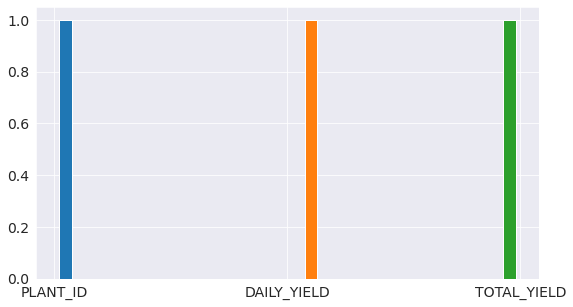In :
``import jovian``
In [ ]:
``jovian.commit()``

## Inferences and Conclusion

*In this note book i have tried to analyze solar power generation system. *i choose this dataset from kaggle.com. *While working on this project, i researched lot of information regarding pandas and plots. *While doing this project i have realized that there is lot more to learn, i am excited to move forward in this journey of becoming a Data Analyst/Scientist. *I have refered to the pandas notebook and visulization notes from zero to pandas project to finish this project, i have done lot research as well to the learn th concepts of pandas and numpy.

In :
``import jovian``
In [ ]:
``jovian.commit()``
In [ ]:
`` ``